# linux 下各个4K区块文件大小测试速度对比 机械、ssd硬盘io性能 128K性价比收益最高 kangle普通（智能）缓存该设置多少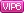成长值: 26320

[LV.Master]伴坛终老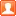发表于 2018/1/26 00:39 | 显示全部楼层 |阅读模式 |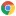Google Chrome 63.0.3239.132|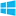Windows 10

1. dd if=/dev/zero of=test bs=4K count=1K oflag=dsync
2. dd if=/dev/zero of=test bs=8K count=1K oflag=dsync
3. dd if=/dev/zero of=test bs=16K count=1K oflag=dsync
4. dd if=/dev/zero of=test bs=32K count=1K oflag=dsync
5. dd if=/dev/zero of=test bs=64K count=1K oflag=dsync
6. dd if=/dev/zero of=test bs=128K count=1K oflag=dsync
7. dd if=/dev/zero of=test bs=256K count=1K oflag=dsync
8. dd if=/dev/zero of=test bs=512K count=1K oflag=dsync
9. dd if=/dev/zero of=test bs=1M count=1K oflag=dsync
10. dd if=/dev/zero of=test bs=2M count=512 oflag=dsync
11. dd if=/dev/zero of=test bs=4M count=256 oflag=dsync
12. dd if=/dev/zero of=test bs=8M count=128 oflag=dsync
13. dd if=/dev/zero of=test bs=16M count=64 oflag=dsync
14. dd if=/dev/zero of=test bs=32M count=32 oflag=dsync
15. dd if=/dev/zero of=test bs=64M count=16 oflag=dsync
16. dd if=/dev/zero of=test bs=128M count=8 oflag=dsync
17. dd if=/dev/zero of=test bs=256M count=4 oflag=dsync

7200转希捷机械硬盘ST1000DM010
 区块 速度 4K 83.2 KB/秒 8K 165 KB/秒 16K 333 KB/秒 32K 668 KB/秒 64K 1.3 MB/秒 128K 2.6 MB/秒 256K 5.2 MB/秒 512K 10.4 MB/秒 1M 17.6 MB/秒 2M 30.0 MB/秒 4M 45.4 MB/秒 8M 67.8 MB/秒 16M 89.3 MB/秒 32M 117 MB/秒 64M 137 MB/秒 128M 148 MB/秒 256M 153 MB/秒

 区块 速度 4K 1.3 MB/秒 8K 2.5 MB/秒 16K 5.3 MB/秒 32K 9.7 MB/秒 64K 18.1 MB/秒 128K 32.9 MB/秒 256K 54.5 MB/秒 512K 84.0 MB/秒 1M 113 MB/秒 2M 141 MB/秒 4M 137 MB/秒 8M 151 MB/秒 16M 164 MB/秒 32M 172 MB/秒 64M 177 MB/秒 128M 179 MB/秒 256M 180 MB/秒

5400转希捷机械硬盘ST1000LM048
 区块 速度 4K 44.7 KB/秒 8K 84.8 KB/秒 16K 164 KB/秒 32K 333 KB/秒 64K 654 KB/秒 128K 1.3 MB/秒 256K 2.4 MB/秒 512K 4.3 MB/秒 1M 7.3 MB/秒 2M 12.3 MB/秒 4M 20.0 MB/秒 8M 29.7 MB/秒 16M 46.1 MB/秒 32M 62.7 MB/秒 64M 77.0 MB/秒 128M 78.4 MB/秒 256M 68.4 MB/秒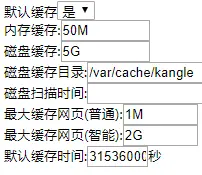本版积分规则 回帖后跳转到最后一页

GMT+8, 2021/1/26 21:11 , Processed in 0.195061 second(s), 22 queries , MemCache On.